## Open tubes

In an open tube, the standing wave of the lowest possible frequency for that particular length of tube (in other words, the fundamental) has antinodes at each end and a node in the centre. This means that an open tube is one-half wavelength long. The fundamental frequency (f1) is thus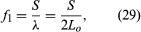where Lo is the length of the open tube. The standing wave of each successive harmonic has one additional loop, as shown by n = 2 and n = 3 in Figure 6. The wavelength (λn) of each successive standing wave is calculated as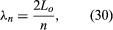and the frequency (fn) as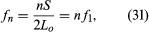just as in the case of the stretched string.

## Closed tubes

The end conditions of a closed tube create a node at the closed end and an antinode at the open end, so that the length of a closed tube (Lc) is one-quarter of a wavelength. For this reason, the length of the closed tubes represented in Figure 6 is one-half that of the open tubes, so that both open and closed tubes produce the same fundamental frequency. In addition, the boundary conditions of a closed tube allow only an odd number of quarter-wavelengths to occupy any given length, so that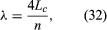where only odd n are allowed. Thus, the frequencies of standing waves in a closed tube include only the odd harmonics,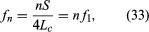where values for n are odd integers only.

## Measuring techniques

A dramatic device used to “observe” the motion of air in a standing wave is the Kundt’s tube. Cork dust is placed on the bottom of this tube, and a standing wave is created. A standing wave in a Kundt’s tube consists of a complex series of small cell oscillations, an example of which is illustrated in Figure 7. The air is set in motion, and the vortex motion of the air cells blows the cork dust into small piles, forming a striation pattern. This pattern is very clear and strong at the velocity antinodes of the standing wave, but it disappears at the locations of nodal points. Alternating locations of nodes and antinodes are thus readily observed using this technique.

Under actual conditions, a node is located exactly at the closed end of a tube, but the antinode, owing to the way a wave reflects when it hits the open end, is actually out past the end of the tube by a small distance known as the end correction. The end correction depends primarily on the radius of the tube: it is approximately equal to 0.6 times the radius of an unflanged tube and 0.82 times the radius of a flanged tube. The effective length of the tube, which must be assumed for the value of L in the equations above, incorporates the end correction.

An important feature of this discussion of standing waves in air columns is that the terms node and antinode refer to the places in the vibrating medium where there is zero and maximum displacement or velocity. Many textbooks and reference works use illustrations in which the wave drawn in a tube represents pressure rather than velocity or displacement. In this case, all the nodes and the antinodes are the reverse of those shown in Figure 6—that is, a pressure node (corresponding to a displacement or velocity antinode) occurs at the open end of a tube, while a pressure antinode (corresponding to a displacement or velocity node) occurs at the closed end. Because most microphones respond to changes in pressure, this type of representation may be more useful when discussing experimental observations involving the use of microphones.

## In solid rods

A thin metal rod can sustain longitudinal vibrations in much the same way as an air column. The ends of a rod, when free, act as antinodes, while any point at which the rod is held becomes a node, so that the representation of their standing waves is identical to that of an open tube. Such standing waves can be activated by sharply striking the end of the rod with a hard object or by scraping the rod with a cloth or with fingers coated with resin. The harmonic frequencies are then given by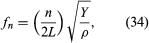where n is the harmonic number, Y is the Young’s modulus (as described above in Plane waves: The speed of sound: In solids), and ρ is the density of the material. This type of standing wave was used by Ernst Chladni in determining the speed of sound in metals.

## In nonharmonic systems

The resonant systems described above have a series of standing-wave resonances that vibrate at the frequencies of the overtone series, but there are several systems whose resonances are not so simply related.

Sound

## External Websites

Britannica Websites
Articles from Britannica Encyclopedias for elementary and high school students.

## Article Contributors

×
Britannica presents a time-travelling voice experience